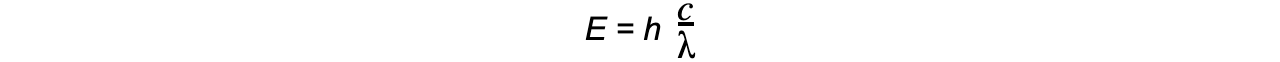# CIE A Level Physics复习笔记22.1.1 The Photon

### The Particle Nature of Light

• In classical wave theory, electromagnetic (EM) radiation is assumed to behave as a wave
• This is demonstrated by the fact EM radiation exhibits phenomena such as diffraction and interference
• However, experiments from the last century, such as the photoelectric effect and atomic line spectra, can only be explained if EM radiation is assumed to behave as particles
• These experiments have formed the basis of quantum theory, which will be explored in detail in this section

### The Photon

• Photons are fundamental particles which make up all forms of electromagnetic radiation
• A photon is a massless “packet” or a “quantum” of electromagnetic energy
• What this means is that the energy is not transferred continuously, but as discrete packets of energy
• In other words, each photon carries a specific amount of energy, and transfers this energy all in one go, rather than supplying a consistent amount of energy

#### Exam Tip

Make sure you learn the definition for a photon: discrete quantity / packet / quantum of electromagnetic energy are all acceptable definitions

### Calculating Photon Energy

• The energy of a photon can be calculated using the formula:

E = hf

• Using the wave equation, energy can also be equal to:• Where:
• E = energy of the photon (J)
• h = Planck's constant (J s)
• c = the speed of light (m s-1)
• f = frequency in Hertz (Hz)
• λ = wavelength (m)
• This equation tells us:
• The higher the frequency of EM radiation, the higher the energy of the photon
• The energy of a photon is inversely proportional to the wavelength
• A long-wavelength photon of light has a lower energy than a shorter-wavelength photon

#### Worked Example

Light of wavelength 490 nm is incident normally on a surface, as shown in the diagram.The power of the light is 3.6 mW. The light is completely absorbed by the surface.Calculate the number of photons incident on the surface in 2.0 s.

Step 1: Write down the known quantities

Wavelength, λ = 490 nm = 490 × 10-9 m

Power, P = 3.6 mW = 3.6 × 10-3 W

Time, t = 2.0 s

Step 2: Write the equations for wave speed and photon energyStep 3: Calculate the energy of one photonStep 4: Calculate the number of photons hitting the surface every secondStep 5: Calculate the number of photons that hit the surface in 2 s

(8.9 × 1015) × 2 = 1.8 × 1016

#### Exam Tip

The values of Planck’s constant and the speed of light will always be given to you in an exam, however, it helps to memorise them to speed up calculation questions!

### Photon Momentum

• Einstein showed that a photon travelling in a vacuum has momentum, despite it having no mass
• The momentum (p) of a photon is related to its energy (E) by the equation:• Where c is the speed of light

#### Worked Example

A 5.0 mW laser beam is incident normally on a fixed metal plate. The cross-sectional area of the beam is 8.0 × 10-6 m2. The light from the laser has frequency 5.6 × 1014 Hz.Assuming that all the photons are absorbed by the plate, calculate the momentum of the photon, and the pressure exerted by the laser beam on the metal plate.

Step 1: Write down the known quantities

Power, P = 5.0 mW = 5.0 × 10-3 W

Frequency, f = 5.6 × 1014 Hz

Cross-sectional area, A = 8.0 × 10-6 m2

Step 2: Write the equations for photon energy and momentum

photon energy:   E = hfStep 3: Calculate the photon momentumStep 4: Calculate the number of photons incident on the plate every secondStep 5: Calculate the force exerted on the plate in a 1.0 s time interval

Force  =  rate of change of momentum

= number of photons per second × momentum of each photon

= (1.35 × 1016) × (1.24 × 10-27)

= 1.67 × 10-11 N

Step 6: Calculate the pressure